# Engineering Mechanics - Planar Kinematics of a Rigid Body (PKRB)

### Exercise :: Planar Kinematics of a Rigid Body (PKRB) - General Questions

• Planar Kinematics of a Rigid Body (PKRB) - General Questions
6.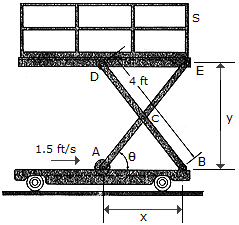The scaffold S is raised hydraulically by moving the roller at A towards the pin at B. If A is approaching B with a speed of 1.5 ft/s, determine the speed at which the platform is rising as a function of. Each link is pin-connected at its midpoint and end points and has a length of 4 ft.

 A. vS = 2.67 sin2ft/s B. vS = 1.500 cotft/s C. vS = 1.500 tanft/s D. vS = 2.67 cosft/s

Explanation:

No answer description available for this question. Let us discuss.

7.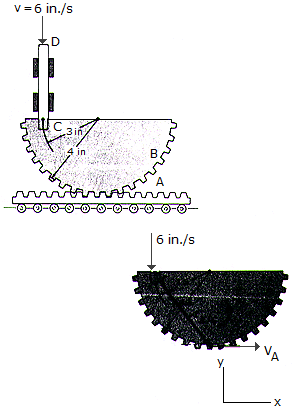If rod CD has a downward velocity of 6in/s at the instant shown, determine the velocity of the gear rack A at this instant. The rod is pinned at C to gear B.

 A. vA = 6.00 in./s B. vA = 8.00 in./s C. vA = 4.50 in./s D. vA = 3.38 in./s

Explanation:

No answer description available for this question. Let us discuss.

8.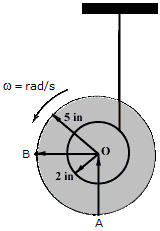As the cord unravels from the wheel's inner hub, the wheel is rotating at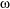= 2 rad/s at the instant shown. Determine the magnitudes of the velocities of point A and B.

 A. vA = 10.77 in./s, vB = 10.00 in./s B. vA = 10.00 in./s, vB = 10.00 in./s C. vA = 10.77 in./s, vB = 14.00 in./s D. vA = 4.00 in./s, vB = 14.00 in./s

Explanation:

No answer description available for this question. Let us discuss.

9.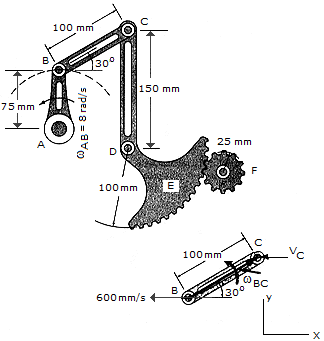The rotation of link AB creates an oscillating movement of gear F. If AB has an angular velocity ofAB = 8 rad/s, determine the angular velocity of gear F at the instant shown. Gear E is a part of arm CD and pinned at D to a fixed point.

 A.F = 26.7 rad/s B.F = 16.00 rad/s C.F = 32.0 rad/s D.F = 27.7 rad/s

Explanation:

No answer description available for this question. Let us discuss.

10.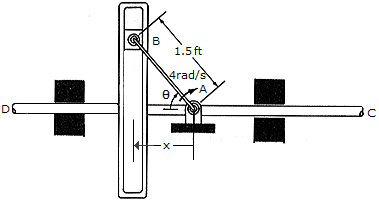The mechanism is used to convert the constant circular motion of rod AB into translating motion of rod CD. Compute the velocity and acceleration of CD for any angleof AB.

 A. vCD = 6 sinft/s, aCD = 24 cos2 ft/s2 B. vCD = 1.5 sinft/s, aCD = 1.5 cos2 ft/s2 C. vCD = 6 sinft/s, aCD = 0 D. vCD = 6 cosft/s, aCD = 0